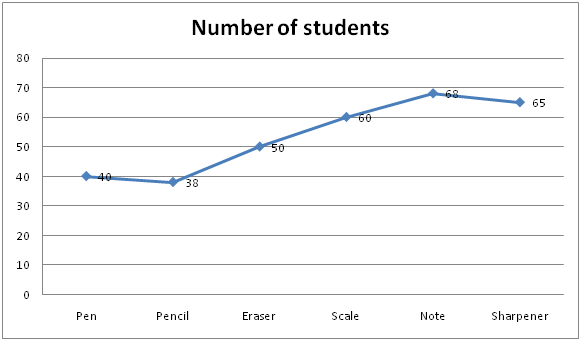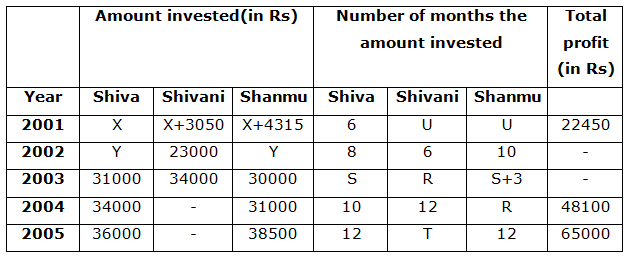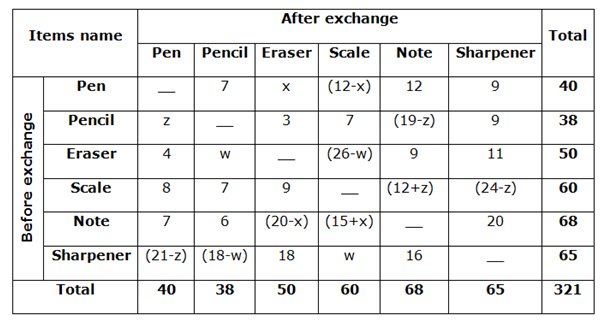# SBI PO Quantitative Aptitude Questions 2019 (Day-11) High Level New Pattern

SBI PO 2019 Notification is about to come and it is the most awaited exam among the aspirants. We all know that new pattern questions are introducing every year in the SBI PO exam. Further, the questions are getting tougher and beyond the level of the candidate’s expectations.

Our IBPS Guide is providing High-Level New Pattern Quantitative Aptitude Questions for SBI PO 2019 so the aspirants can practice it on a daily basis. These questions are framed by our skilled experts after understanding your needs thoroughly. Aspirants can practice these high-level questions daily to familiarize with the exact exam pattern. We wish that your rigorous preparation leads you to a successful target of becoming SBI PO.

#### “Be not afraid of growing slowly; be afraid only of standing still”

[WpProQuiz 4891]

Directions (1 – 5): Read the following information carefully and answer the given questions

In a class of 321 students 321 stationary items are distributed such that every student gets exactly 1 item. There are six different items that are distributed among these students namely Pen, Pencil, Erase, Scale, Note and Sharpener. The following line – graph provides information about the number of students who received Pen, Pencil, Erase, Scale, Note and Sharpener.The students exchanged the items among themselves and after they stopped further exchanging, it was noted that no student had the same item that he/she had earlier. Even after the exchange every students has exactly one item.

• Among the students that originally had pen, the number of students now having Pencil, Note and Sharpener is 7, 12 and 9 respectively.
• Among the students that originally had Pencil, the numbers of students now having Eraser and scale is 3 and 9 respectively.
• Among the students that originally had Eraser, the number of students now having Pen, Note and Sharpener is 4, 9, and 11 respectively.
• Among the students that originally had Scale, the number of students now having Pen, Pencil, Eraser is 8, 7 and 9 respectively.
• Among the students that originally had Note, the number of students now having Pen, Pencil and Sharpener is 7, 6 and 20 respectively.
• Among the students that originally had Sharpener, the number of students now having Eraser and Note is 18 and 16 respectively.

1) Among the students that originally had Pen, at least how many students must now have Scale?

a) 5

b) 3

c) 2

d) 1

e) 6

2) If out of the students that originally had Pen, the number of students now having Scale is 8, then find out of the students that now have Eraser?

a) 3

b) 2

c) 4

d) 1

e) 5

3) If among the students that originally had Note, the number of students now having Eraser is 1 more than the number of students now having Scale, then find the difference between the number of the students that now have Sharpener and Scale

a) 4

b) 3

c) 5

d) 6

e) 12

4) If among the students that originally had Sharpener, the number of students now having Eraser is twice the number of students now having Pen, then find out among the students that now have Scale which is one student more than the number of students that now have Pen?

a) 10

b) 9

c) 16

d) 15

e) 18

5) If among the students that originally had Pencil, the number of students now having Note is same as the number of students now having Scale, then find among the students that originally had Sharpener, the number of students now having pen

a) 7

b) 9

c) 8

d) 6

e) 10

Directions (6 – 10): Three partners Shiva, Shivani and Shanmu invested different amounts in different years for different time periods and shared their profit from total profit given as follows.6) If Shivani’s investment in 2004 is Rs 8000 less than that of Shiva’s investment in the same year and Shanmu’s profit in 2004 is Rs 15500 then find for how many months did Shanmu invest his money in the year 2004.

a) 9 months

b) 10 months

c) 6 months

d) 12 months

e) 11 months

7) Find the profit of Shanmu in 2003, if the profit of Shiva and Shivani in the same year is Rs 13950 and Rs 17000 respectively. (R = 10 months)

a) Rs 18000

b) Rs 15580

c) Rs 17000

d) Rs 17500

e) Rs 16000

8) In 2001, if the difference between the profit shares of Shivani and Shanmu is Rs 550 and profit share of Shiva is Rs 7500, then find total investment of all of three.

a) Rs 42680

b) Rs 47280

c) Rs 43270

d) Rs 47908

e) Rs 47895

9) Shivani’s investment in 2005 is 32% greater than that of Shiva in same year and the value of T is 12 months. If profit of Shivani is Rs 23760, then what is the profit of Shanmu?

a) Rs 15820

b) Rs 16520

c) Rs 19250

d) Rs 15880

e) Rs 18580

10) In 2002, profit of Shiva and Shanmu together is Rs 16400 and the difference between their profit shares is Rs 1200. Find the total profit in that year.

a) Rs 27920

b) Rs 24650

c) Rs 31620

d) Rs 19720

e) Cannot be determined

Directions (1 – 5):From the above table, out of the students that originally had Pen, the number of students now having Scale is ‘12 – x’. Maximum possible value of x is 11.

Therefore minimum possible value of 12 – x

= 12 – 11= 1

From the above table, out of the students that originally had Pen, the number of students now having Scale is 8 = (12-x)

8 = 12 –x

= > x = 4

Out of the students that originally had Pen, the number of students now having Eraser is 4.

Among the students that originally had Note, the number of students now having Scale is a

Among the students that originally had Note, the number of students now having Eraser is b

From the above table, among the students that originally had Note, the total number of students now having Eraser and Scale is

(68 – 35) = 33 = (a + b) — (1)

= > (b – a) = 1 —- (2)

Solve the above equation (1) and (2), we get

= > a = 34/2 = 17

= > b = 16

Required difference = 20 – 17 = 3

Among the students that originally had Sharpener, the number of students now having Eraser is 18

Among the students that originally had Sharpener, the number of students now having Pen be (18/2) = 9

Among the students that originally had Sharpener, the number of students now having Scale = 9 + 1 = 10

From the above table,

Among the students that originally had Pencil, the number of students now having Note = among the students that originally had Pencil, the number of students now having Scale = 7

Among the students that originally had Pencil, the number of students now having Pen = 38 – (3+7+9+7) = 38 – 26 = 12 = z

Among the students that originally had Sharpener, the number of students now having pen = (21 – z)

= 21 – 12 = 9

Direction (6-10) :

Shivani’s investment in 2004=34000-8000= Rs 26000

Since Shanmu’s profit is Rs 15500, then total profit of Shiva and Shivani together =48100-15500=Rs 32600

Then 15500/32600= (31000*R)/(34000*10+26000*12)

155/326=31*R/652

R=10 months.

From above question, we have R= 10 months.

Then 13950/17000= (31000*S)/(34000*10)

S=9 months.

Let profit of Shanmu be Rs a.

Then a/17000= (30000*12)/ (34000*10)

a=Rs 18000

(i.e) Profit of Shanmu=Rs 18000

From table, total profit of all three partners in 2001=22450

Given that profit share of Shiva=Rs 7500

Then total profit of Shivani and Shanmu= 22450-7500=Rs 14950

Since the difference between the profit shares of Shivani and Shanmu is Rs 550,

Profit share of Shivani=Rs 7200 and

Profit share of Shanmu=Rs 7750 [If the investment is more, then the share of profit also more than that of others]

Then (X+3050)/(X+4315) =7200/7750

(X+3050)/(X+4315) = 144/155

155x + 472750 = 144x + 621360

11x = 148610

X= Rs 13510

Thus, total investment of all three partners=13510+13510+3050+13510+4315

=Rs 47895

Shivani’s investment in 2005=36000*(132/100) =Rs 47520

Let the profit of Shanmu be P,

Then, according to the question,

(47520/38500)=23760/P [Here period is equal, so, the ratio of profit = Ratio of investment]

P= (23760*38500)/47520

P=Rs 19250

Without knowing the shares of investments of Shiva and Shanmu, We cannot find the total profit of all three partners in that year.

### Click Here for SBI PO Pre 2019 High-Quality Mocks Exactly on SBI Standard

Daily Practice Test Schedule | Good Luck

 Topic Daily Publishing Time Daily News Papers & Editorials 8.00 AM Current Affairs Quiz 9.00 AM Current Affairs Quiz (Hindi) 9.30 AM NIACL AO Prelims – Reasoning 10.00 AM NIACL AO Prelims – Quantitative Aptitude 11.00 AM Vocabulary (Based on The Hindu) 12.00 PM NIACL AO Prelims – English Language 1.00 PM SSC Practice Questions (Reasoning/Quantitative aptitude) 2.00 PM IBPS Clerk – GK Questions 3.00 PM SSC Practice Questions (English/General Knowledge) 4.00 PM Daily Current Affairs Updates 5.00 PM SBI PO/IBPS Clerk Mains – Reasoning 6.00 PM SBI PO/IBPS Clerk Mains – Quantitative Aptitude 7.00 PM SBI PO/IBPS Clerk Mains – English Language 8.00 PM